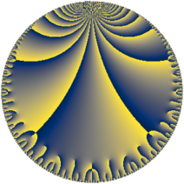# Properties

 Label 96.10.cLevel $96$ Weight $10$ Character orbit 96.c Rep. character $\chi_{96}(95,\cdot)$ Character field $\Q$ Dimension $36$ Newform subspaces $1$ Sturm bound $160$ Trace bound $0$

# Related objects

## Defining parameters

 Level: $$N$$ $$=$$ $$96 = 2^{5} \cdot 3$$ Weight: $$k$$ $$=$$ $$10$$ Character orbit: $$[\chi]$$ $$=$$ 96.c (of order $$2$$ and degree $$1$$) Character conductor: $$\operatorname{cond}(\chi)$$ $$=$$ $$12$$ Character field: $$\Q$$ Newform subspaces: $$1$$ Sturm bound: $$160$$ Trace bound: $$0$$

## Dimensions

The following table gives the dimensions of various subspaces of $$M_{10}(96, [\chi])$$.

Total New Old
Modular forms 152 36 116
Cusp forms 136 36 100
Eisenstein series 16 0 16

## Trace form

 $$36 q - 27740 q^{9} + O(q^{10})$$ $$36 q - 27740 q^{9} + 194616 q^{13} + 634168 q^{21} - 13299948 q^{25} + 9661040 q^{33} - 16866216 q^{37} + 35926304 q^{45} - 152499348 q^{49} + 261857496 q^{57} - 497603016 q^{61} - 1087284512 q^{69} - 840635352 q^{73} - 1155854332 q^{81} + 348272640 q^{85} - 1080661448 q^{93} + 820514664 q^{97} + O(q^{100})$$

## Decomposition of $$S_{10}^{\mathrm{new}}(96, [\chi])$$ into newform subspaces

Label Dim $A$ Field CM Traces $q$-expansion
$a_{2}$ $a_{3}$ $a_{5}$ $a_{7}$
96.10.c.a $36$ $49.443$ None $$0$$ $$0$$ $$0$$ $$0$$

## Decomposition of $$S_{10}^{\mathrm{old}}(96, [\chi])$$ into lower level spaces

$$S_{10}^{\mathrm{old}}(96, [\chi]) \cong$$ $$S_{10}^{\mathrm{new}}(12, [\chi])$$$$^{\oplus 4}$$$$\oplus$$$$S_{10}^{\mathrm{new}}(48, [\chi])$$$$^{\oplus 2}$$